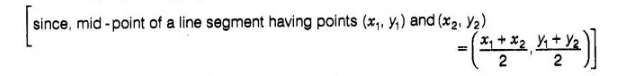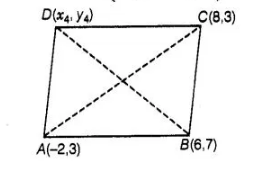# The fourth vertex D of a parallelogram ABCD

Question:

The fourth vertex D of a parallelogram ABCD whose three vertices are A(- 2, 3), B(6, 7) and C(8, 3) is

(a) (0,1)

(b) (0,-1)

(c) (-1,0)

(d) (1,0)

Solution:

(b) Let the fourth vertex of parallelogram, D≡(x4 ,y4) and L, M be the middle points of AC and BD, respectively,

Then, $L=\left(\frac{-2+8}{2}, \frac{3+3}{2}\right)=(3,3)$and $M=\left(\frac{6+x_{4}}{2}, \frac{7+y_{4}}{2}\right)$Since, ABCD is a parallelogram, therefore diagonals AC and BD will bisect each other. Hence, L and M are the same points.

$\therefore$ $3=\frac{6+x_{4}}{2}$ and $3=\frac{7+y_{4}}{2}$

$\Rightarrow \quad 6=6+x_{4}$ and $6=7+y_{4}$

$\Rightarrow \quad x_{4}=0 \quad$ and $y_{4}=6-7$

$\therefore \quad x_{4}=0 \quad$ and $y_{4}=-1$

Hence, the fourth vertex of parallelogram is D s (x4, y4) s (0,-1).